# 一行 Python 代码能实现什么丧心病狂的功能？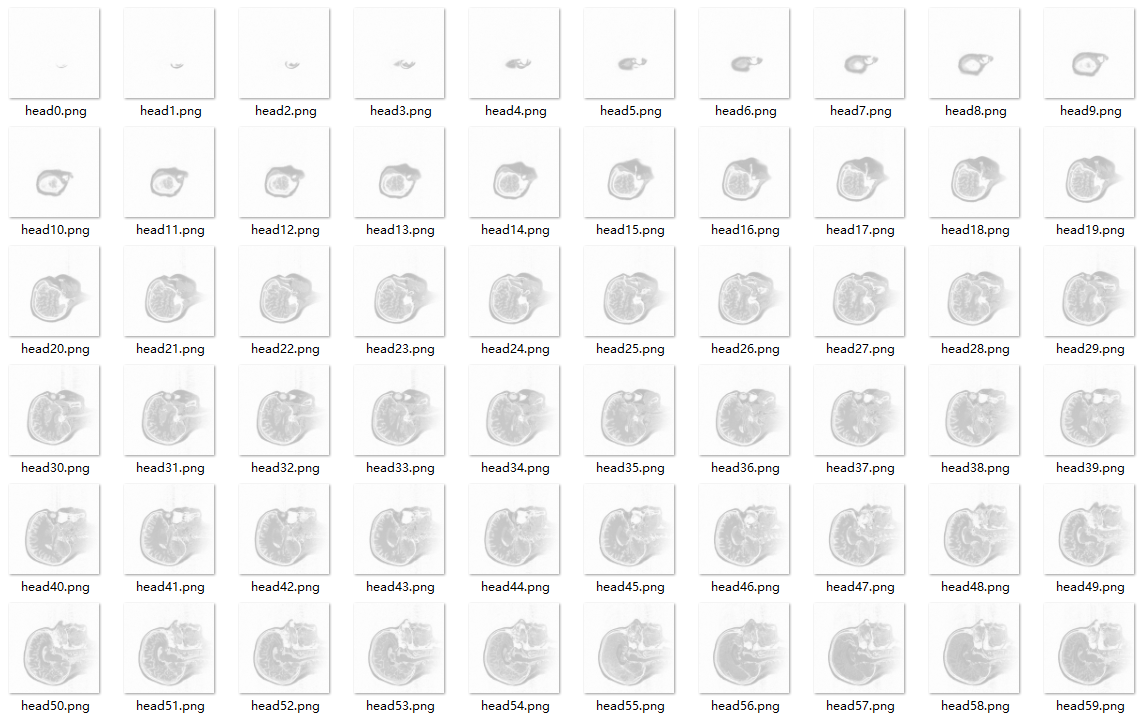import numpy as np
from PIL import Image


data = np.stack([np.array(Image.open('head%d.png'%i)) for i in range(109)], axis=0)


data = list()
for i in range(109):
img = Image.open('head%d.png'%i)
img = np.array(img)
data.append(img)
data = np.stack(data, axis=0)


#### 1. 一行代码打印乘法口诀

print('\n'.join([' '.join(["%2s x%2s = %2s"%(j,i,i*j) for j in range(1,i+1)]) for i in range(1,10)]))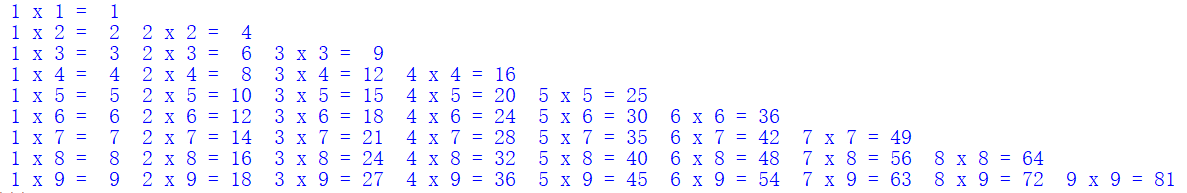#### 2. 一行代码打印迷宫

print(''.join(__import__('random').choice('\u2571\u2572') for i in range(50*24)))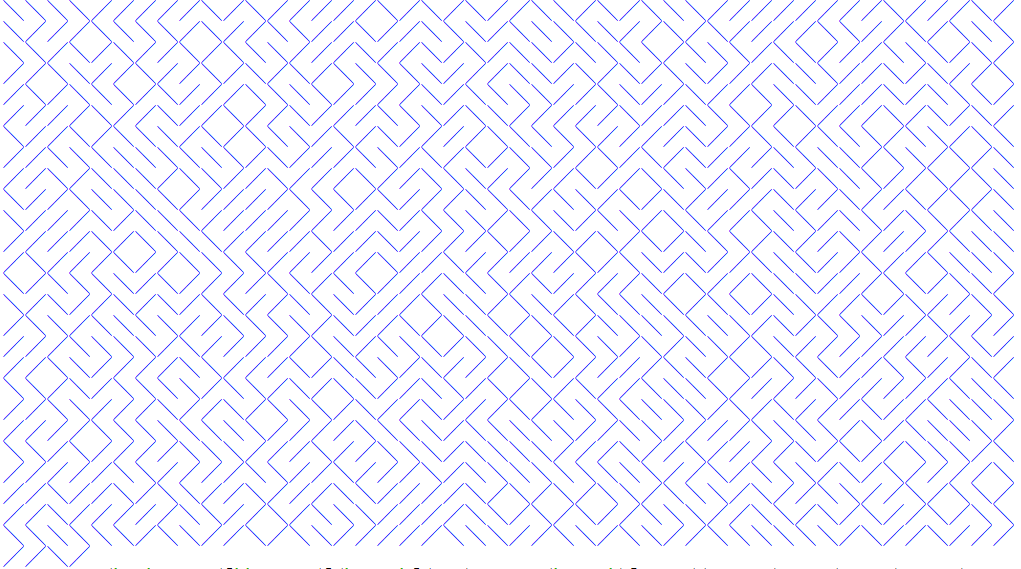#### 3. 一行代码表白爱情

print('\n'.join([''.join([('Love'[(x-y) % len('Love')] if ((x*0.05)**2+(y*0.1)**2-1)**3-(x*0.05)**2*(y*0.1)**3 <= 0else' ') for x in range(-30, 30)]) for y in range(30, -30, -1)]))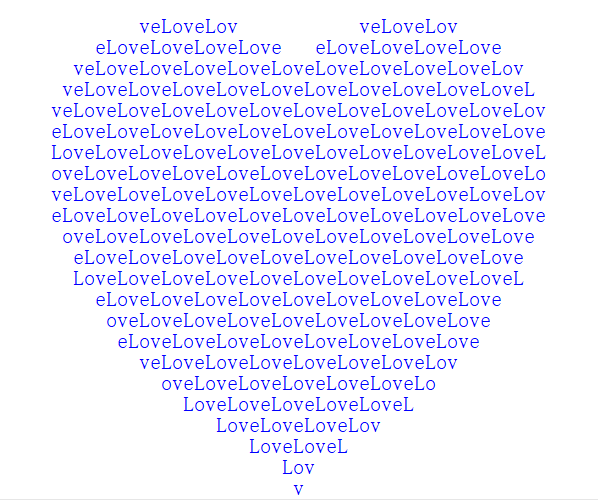#### 4. 一行代码打印小龟龟

print('\n'.join([''.join(['*' if abs((lambda a:lambda z,c,n:a(a,z,c,n))(lambda s,z,c,n:z if n==0 else s(s,z*z+c,c,n-1))(0,0.02*x+0.05j*y,40))<2 else ' ' for x in range(-80,20)]) for y in range(-20,20)]))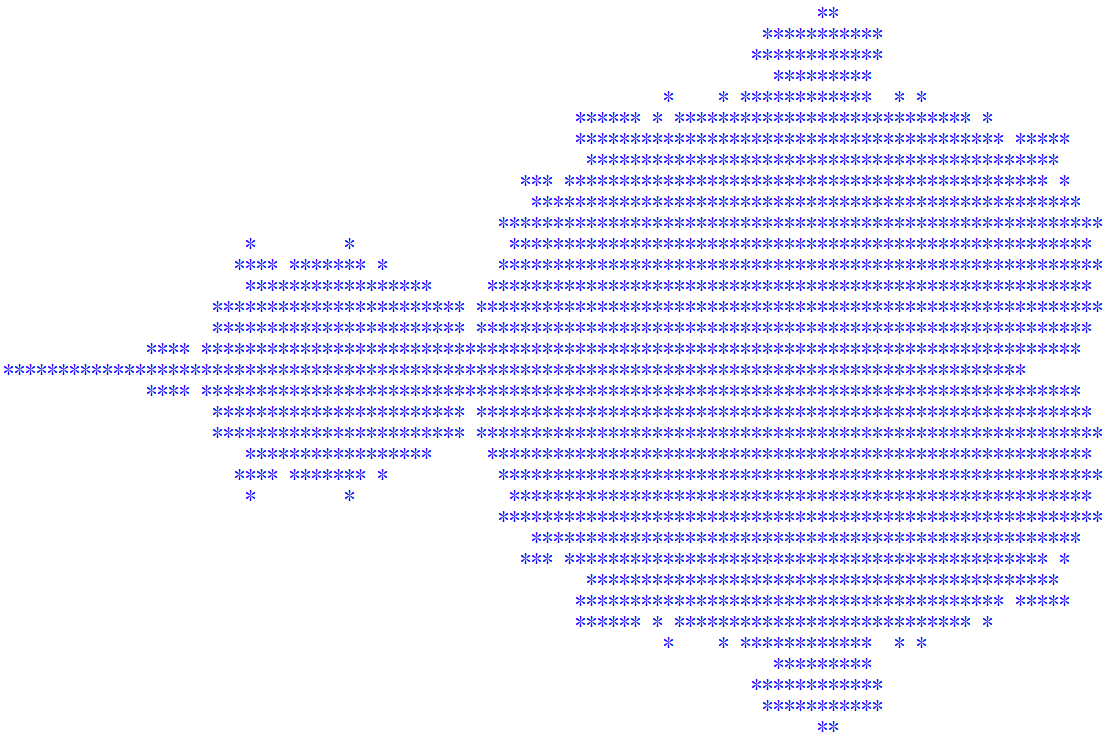#### 后记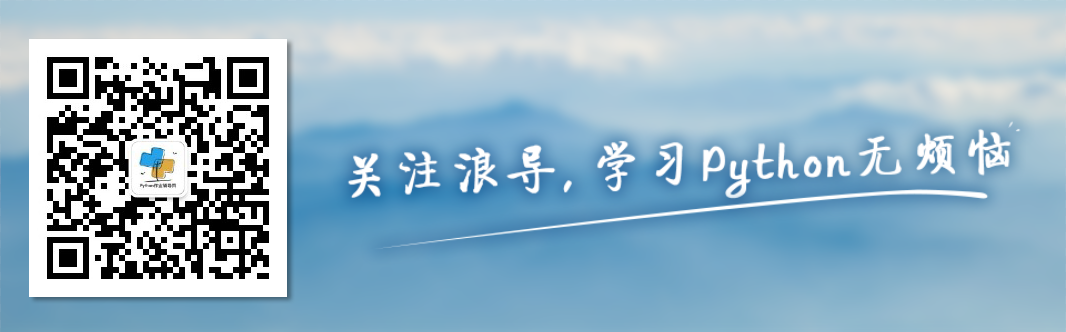05-041万+12-124万+
02-244447
05-021万+
08-026万+
12-2019
11-29582
01-13371
02-239579
08-307万+
08-277976
12-30427
09-0582
08-169544
04-301万+
10-021万+
01-18190
07-17206
01-13396
©️2020 CSDN 皮肤主题: 代码科技 设计师:Amelia_0503点击重新获取扫码支付1.余额是钱包充值的虚拟货币，按照1:1的比例进行支付金额的抵扣。
2.余额无法直接购买下载，可以购买VIP、C币套餐、付费专栏及课程。余额充值